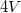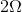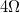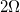Test: AP Physics B

 1 A student assembles a circuit made up of a voltage source and two resistors. All three circuit elements are connected in parallel. The voltage across the voltage source isand the resistance of the resistors areandrespectively. Which of the following is true of this circuit?

The current through theresistor will be less than the current through theresistor

The voltage across theresistor will equal the voltage across theresistor

The current through theresistor will equal the current through theresistor

The voltage across theresistor will be less than the voltage across theresistor

1/2 questions

0%

Access results and powerful study features!

Take 15 seconds to create an account.